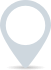# Principal

Principal refers to the original sum of money that is invested, borrowed, or lent. It is the initial amount before any interest, gains, or losses are factored in. In various financial contexts, “principal” can have different meanings:

1. Investments: In investments, the principal is the initial amount of money that is invested. For example, if you invest £1,000 in a stock, the £1,000 is the principal.

2. Loans and Debt: When you borrow money, the principal is the original amount you borrowed. For example, if you take out a £5,000 loan, the £5,000 is the principal amount.

3. Bonds: In the context of bonds, the principal is the face value of the bond. It is the amount that will be repaid to the bondholder at the bond’s maturity date.

4. Mortgages: In a personal or commercial mortgage loan, the principal is the initial amount borrowed to purchase a home. Over time, as you make mortgage payments, a portion of the payment goes towards reducing the principal, while the rest covers interest and possibly other costs.

5. Savings and Investments: In savings accounts or investment products, the principal refers to the initial amount of money you deposit or invest. Any interest or returns earned are typically calculated based on this initial sum.

6. Derivatives: In the context of derivatives, such as options or futures contracts, the principal can refer to the amount of money required to establish a position. For example, in a futures contract, the principal is the amount of money needed to enter into the contract.

7. Real Estate: In real estate transactions, the principal refers to the initial purchase price of a property.

It’s important to note that the principal amount does not include any interest or returns earned over time. In the case of loans, the interest is the cost of borrowing and is calculated based on the principal. In investments, returns are typically earned on the principal amount. Understanding the concept of principal is fundamental in various financial transactions and calculations.### Clever finance tips and the latest news

#### delivered to your inbox, every week